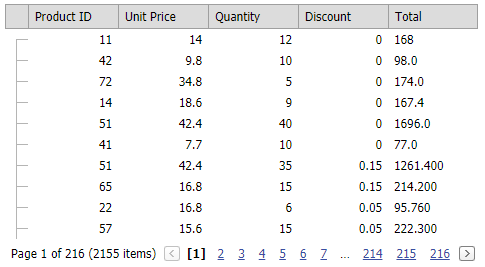The requested page is not available for the requested platform. You are viewing the content for Default platform.

19.2
19.1
18.2
18.1
17.2

# TreeListCustomUnboundColumnDataEventArgs.Value Property

Gets the edit value of the cell currently being processed.

Namespace: DevExpress.Web.ASPxTreeList

Assembly: DevExpress.Web.ASPxTreeList.v19.2.dll

## Declaration

``public object Value { get; set; }``
``Public Property Value As Object``

## Property Value

Type Description
Object

An object that represents the cell's edit value.

## Examples

Assume that ASPxTreeList is bound to an "Order Details" data table (NWind data base), which contains "UnitPrice", "Quantity" and "Discount" fields. There is no field that represents the total sum, as this can be calculated manually as follows: UnitPrice*Quantity*(1-Discount). This example shows how to add an unbound column to the ASPxTreList control to represent the total sum of an order.

The image below shows the result.``````protected void TreeList_CustomUnboundColumnData(object sender, TreeListCustomUnboundColumnDataEventArgs e)
{
if (e.Column.FieldName == "Total")
{
decimal unitPrice = Convert.ToDecimal(e.Node.GetValue("UnitPrice"));
decimal quantity = Convert.ToDecimal(e.Node.GetValue("Quantity"));
decimal discount = Convert.ToDecimal(e.Node.GetValue("Discount"));
e.Value = unitPrice * quantity * (1 - discount);
}
}
``````